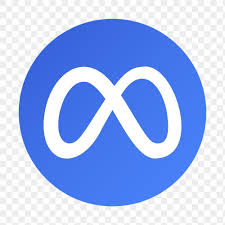## Merge Segments(This question has been seen in the interviews of the following companies: Facebook)

# There's a room with a TV and people are coming in and out to watch it. The TV is on only when there's at least a person in the room.
# For each person that comes in, we record the start and end time. We want to know for how long the TV has been on. In other words:
# Given a list of arrays of time intervals, write a function that calculates the total amount of time covered by the intervals.
# For example:
# input = [(1,4), (2,3)]
# > 3
# input = [(4,6), (1,2)]
# > 3
# input = {{1,4}, {6,8}, {2,4}, {7,9}, {10, 15}}
# > 11

``````
public static int mergeSegments(int[][] segments) {
if(segments.length == 0) return 0;

TreeMap map = new TreeMap<>();

for(int[] s: segments) {

int start, end;
Integer sKey = map.floorKey(s);
if(sKey == null || map.get(sKey) < s) {
start = s;
end = s;
} else {
start = sKey;
end = Math.max(s, map.get(sKey));
}

Integer next = map.higherKey(start);
while(next != null && map.get(next) <= end) {
end = Math.max(map.get(next), end);
map.remove(next);
next = map.higherKey(next);
}
map.put(start, end);
}

int sum = 0;
for(Map.Entry entry: map.entrySet()) {
sum += entry.getValue() - entry.getKey();
}
return sum;
}
``````